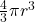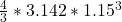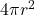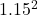## Bacteria vary somewhat in size, but a diameter of 2.3 m is not unusual.A. What would be the volume (in cubic centimeters) of such a bacteriu

Question

Bacteria vary somewhat in size, but a diameter of 2.3 m is not unusual.A. What would be the volume (in cubic centimeters) of such a bacterium, assuming that it is spherical?Express your answer using two significant figures.B. What would be the surface area (in square millimeters) of such a bacterium, assuming that it is spherical?

in progress 0
2 months 2021-07-28T01:40:53+00:00 1 Answers 2 views 0

a) 6.4 x 10^-12 cm^3

b) 17 x 10^-6 mm^2

Explanation:

The complete question is…

Bacteria vary somewhat in size, but a diameter of 2.3 m is not unusual.

A. What would be the volume (in cubic centimeters) of such a bacterium, assuming that it is spherical? Express your answer using two significant figures.

B. What would be the surface area (in square millimeters) of such a bacterium, assuming that it is spherical?

Diameter of the bacteria d = 2.3 μm

Radius r = d/2 = 2.3/2 = 1.15 μm

The shape is assumed to be spherical

a) The volume = volume of a sphere =V == 6.3715 μm^3

1 μm^3 = 10^-12 cm^3

6.3715 μm^3 = 6.3715 x 10^-12 cm^3

==> 6.4 x 10^-12 cm^3

b) The surface area =A = 4 x 3.142 x= 16.62 μm^2

1 μm^2 = 10^-6 cm^2

16.62 μm^2 = 16.62 x 10^-6 mm^2

==> 17 x 10^-6 mm^2